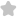The resultant of A and B makes an a...
Question# The resultant of A and B makes an angle a with A and B with B then (A) a JEE/Engineering Exams Physics SolutionShare1524.0 (1 ratings)Alnray the resceltant benols tonards the veetor which ig higher in magnit tucle is If the nagnitude of ( vec{A} ) and ( vec{B} ) will be same then ( 2=beta ). But is suppose ( vec{A} ) has higher magnimole, than ( vec{B} ) then resultant aill bend to nard ( beta ) A and hence ( (alpha1524.0 (1 ratings) Rate SolutionShareQuick and Stepwise Solutions Just click and SendOVER 20 LAKH QUESTIONS ANSWERED Download App for Free